Liquid Measurement Chart - Definition with Examples

The Complete K-5 Math Learning Program Built for Your Child

• 30 Million Kids

Loved by kids and parent worldwide

• 50,000 Schools

Trusted by teachers across schools

• Comprehensive Curriculum

Aligned to Common Core

Liquid Measurement Chart

Liquid Measurement

The liquid measurement is the amount of liquid a vessel contains and its measurement in standard units. We also refer to it as the “capacity” or the “volume” of the vessel.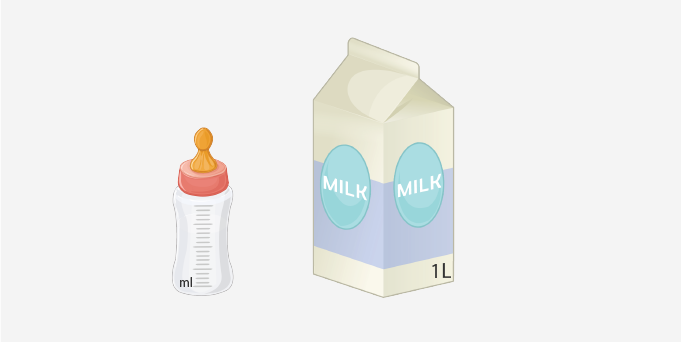Infant milk bottle with measurement markings in milliliter and juice bottle with 1 liter contents.

The different tools used for liquid measurement are:

• Measuring Cup & Pitcher: We commonly use the measuring cup and pitcher with markings in the kitchen for adding liquid ingredients in a measured quantity.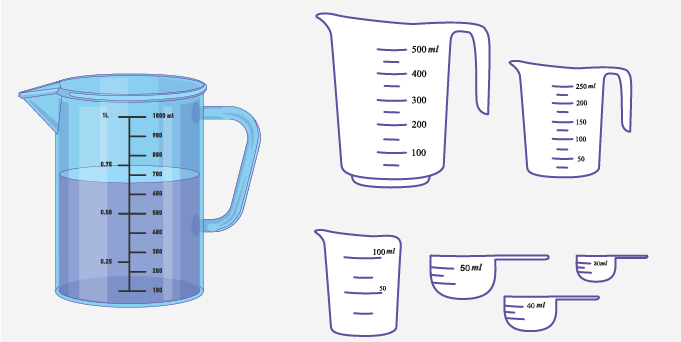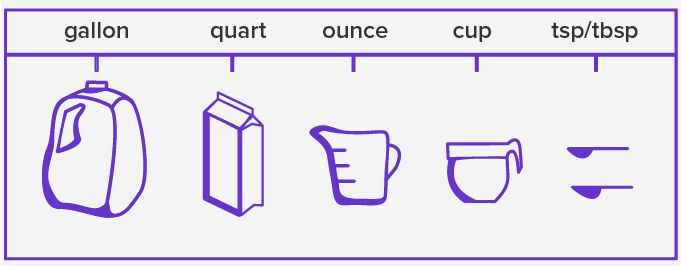Measuring pitcher, cups and spoons with measurement markings in metric and US customary units on the surface.

• Laboratory essentials: The liquid measurement equipment such as beakers, graduated cylinder, test tube, conical flasks are used in laboratories for clinical and scientific purposes. With permanent markings in metric and customary, these types of equipment are useful in measuring liquids with precision for carrying out tests with chemicals and other liquid compounds.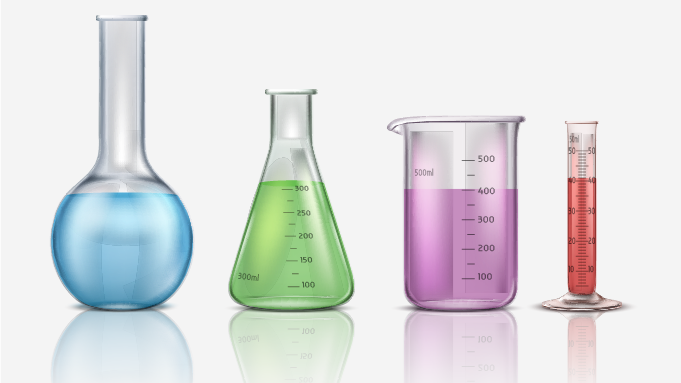Conical flat-bottom flask, beaker and graduated cylinder; liquid measurement equipments used in laboratory markings.

Units of Liquid Measurement

 International System / Metric Units U.S. Customary Units U.K. Imperial Units Milliliter – ml  Centiliter – cl  Liter – l  Kiloliter – kl Fluid ounce – fl. oz.  Cup  Pint  Quart  Liquid Gallon Fluid ounce – fl. oz.  Cup  Pint  Quart  Liquid Gallon

Unit Conversion

To convert the mentioned unit to different units, follow the given conversion steps.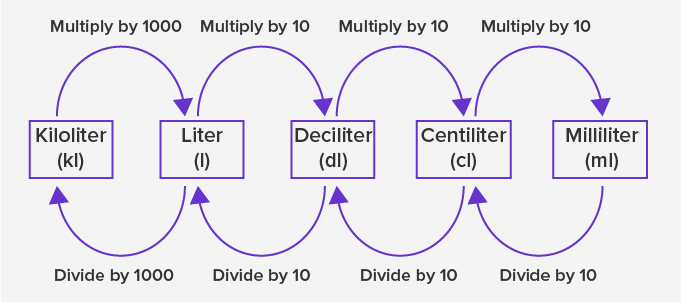Metric unit conversion for different measurements.

Let’s convert the quantities to different units using the conversion table.

 Milliliter Centiliter Liter Kiloliter 5 Liter Multiply by 1000  = 5 × 1000  = 5000 ml Multiply by 100  = 5 × 100  = 500 cl Given in the same unit 5 l Divide by 1000  = 5 ÷ 1000  = 0.005 kl 400 Milliliter Given in the same unit  400ml Divide by 10  = 400 ÷ 10  = 40 cl Divide by 1000  = 400 ÷ 1000  = 0.4 l Divide the value in liter by  1000  = 0.4 ÷ 1000  = 0.0004 kl

We follow the given chart for U.S. customary unit conversions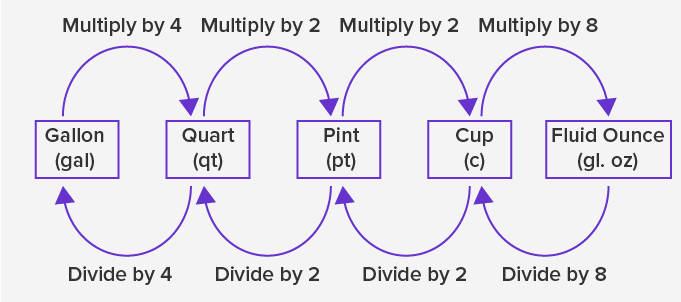customary unit conversion for different measurements.

An example of using the above chart for unit conversion is:

 U.S. Customary Cup Pint Quart Gallon 2000 fl. oz Divide by 8  = 2000 ÷ 8  = 250 c Divide the “cup” by 2  = 250 ÷ 2  = 125 pt Divide the “pint” by 2  = 125 ÷ 2  = 62.5 qt Divide the “quart” by 4  = 62.5 ÷ 4  = 15.62 gal

We follow the given chart for U.K. Imperial unit conversions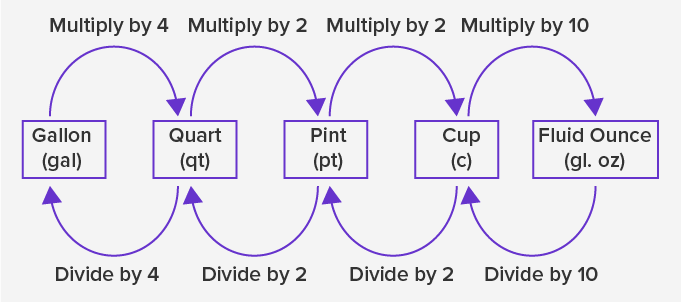Imperial unit conversion for different measurements.

Using the above chart, the conversion of a given capacity in different units is illustrated as follows:

 UK Imperial Units Fluid Ounce Pint Quart Gallon 40 cups Multiply by 10  = 40 × 10  = 400 fl oz. Divide by 2  = 20 ÷ 2  = 10 qt Divide the “pint” by 2  = 20 ÷ 2  = 10 qt Divide the “quart” by 4  = 10 ÷ 4 = 2.25 gal

Application

The liquid measurement units are commercially used for the trade purpose of commodities such as petrol, water, oil, and others. Standardization of measurement units introduced uniformity for global interpretation of mass and volume of liquids.

 Fun Facts Romans used bronze vessels with markings to measure liquids using the unit “sextarius”, equivalent to an imperial pint of 568 ml. Mercury is the only liquid metal. The thickness of a liquid is known as its viscosity. Liquids such as honey, melted chocolate and mayonnaise are more viscous than oil, water, and milk.

Related Math Vocabulary

• Fluids

• Weight of liquids

• Imperial Metrics

• SI Metrics

• Unit conversion for liquid measurement

Won Numerous Awards & Honors